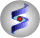ICM Manual v.3.9
by Ruben Abagyan,Eugene Raush and Max Totrov
Nov 28 2023

 Prev ICM Language Reference Chemical Functions Next

The list of functions is expanding. See add column function section for the syntax to use the in a command line. See the GUI/Chemistry menu in ICM-graphics version for different properties that are added through some of these functions. The current list of the functions is the following: E.g. add column t function="MolPSA(mol)" name="PLSA"

• MolWeight(mol ["monoiso"|"aveiso"]) : molecular mass in Dalton, default is "monoiso"
• MolFormula(mol) : chemical composition formula, eg {"C2H6O",..}
• IupacName(mol) : IUPAC names of chemicals from .mol, e.g. {"ethanol",..}
• MolLogP(mol) : Octanol water partition, Log10(C_oct/C_w) from .mol prediction
• MolLogD(mol) : LogD is a log of partition of a chemical compound between the lipid and aqueous phases
• MolLogS(mol) : predicted water solubility, Log10([C]): logarithm of the solubility in moles/liter
• MolPSA(mol) : polar surface area in squared Angstoms
• MolArea(mol) : rarray of predicted total surface areas in square angstroms
• MolVolume(mol) : rarray of predicted volume in cubic angstroms
• MoldHf(mol) : predicted heats of formation in kJ/mol
• pKa_ma(mol) : Calculate pKa the Most Acidic Group
• pKa_mb(mol) : Calculate pKa the Most Basic Group
• DrugLikeness(mol) : predicted drug-likeness values, approx.normal distribution N(0,1.), positive values are more drug-like.
• MolSynth(mol) : chemical synthetic accessibility values [0-1]; values of 1. mean that all fragments are very common, zero value - too many rare or unknown fragments
• Smiles(mol,['unique'|'unique_cistrans'|'asis'|'topology'|'unique_topology']) : SMILES/SMARTS strings. Default - 'unique'.
• Nof_Atoms(mol,[]) : n.of atoms of specified type, e.g. '*'|'H'|'[!H]'|'[#6]'|'[!#6]'|..
• Nof_Molecules(mol) : Number of individual molecules in .mol drawings
• Nof_Fragments(mol,) n.of specified fragments, e.g. Nof_Fragments(mol,'CCC')
• Nof_Chirals(mol,['any'|'left'|'right'|'racemic']) : n.of chiral centers, R,S,or (RS) from .mol
• Max_Ring_Size(mol) : Largest independent ring size from .mol
• Min_Ring_Size(mol) : Smallest independent ring size from .mol
• Max_Fused_Rings(mol,[(no)]) : n.of elementary rings in the largest fused ring
• Nof_RotB(mol) : n.of freely rotatable bonds
• Nof_HBA(mol,[(yes)] ) : n.of hydrogen bond acceptors
• Nof_HBD(mol) : n.of hydrogen bond donors
• ToxScore(mol,,) : bad score is ≥ 1.5
• predCovfunc(mol) : predicts covalent function and adds two columns: MolCovalent, and MolProdrug
Example of how to use the functions:
```
add column t Chemical({"CCO","CCCCO","CO"}) {"ethanol","propanol","methanol"} name={"mol","name"} #make (or read) a chemical table.
```

Direct Icm built-in-functions, e.g.:

• Descriptor(,number,atom) : Atom counts
• Descriptor(,number,bond) : Bond counts
• Descriptor(,number,topology) : Topological, connectivity and shape indices
• Find(,problem) : unwanted or reactive chemical functionality from .mol
Direct Icm shell-script-functions, e.g. predCovfunc( X ):

Functions using Molscreen models in \$ICMHOME/models/ folder (alternatively, the full path to the model-file location needs to be specified).

• predictModelsCol(mol,'mcpCACO2') (MolCACO2 column) : Predict CACO-2 Permeability LogPapp in cm/sec, a value >-5. indicates high permeability, <-6. indicates low permeability
• predictModelsCol(mol,'mcphERGclass') MolHERG : Predict hERG inhibition, a value >0.5 indicates high probability of being a hERG inhibitor
• predictModelsCol(mol,'mcpLD50') MolLD50 : Predict Log LD50 in mg/kg, a value of <0 indicates 1mg/kg high toxicity, 2 indicates 100mg/kg low toxicity
• predictModelsCol(mol,'mcpLogHALFLIFE') MolLogHALFLIFE : Predict Log10 Half Life in Human Plasma in hour, a value >1. indicates half life > 10 hours; Also output half life in plasma and microsome in hours
• predictModelsCol(mol,'mcpLogPERME') MolLogPERME : Predict Log Permeability of PAMPA, CACO2 in cm/sec, a value >-5. indicates high permeability, <-6. indicates low permeability; Efflux Ratio of CACO2 >2. indicates active efflux
• predictModelsCol(mol,'mcpPAINS') MolPAINS : Predict Pan Assay Interference Compound (PAINS), a value >0.5 indicates high probability of being a PAINS Compound
• predictModelsCol(mol,'mcpPAMPA') MolPAMPA : Predict PAMPA Permeability LogPapp in cm/sec, a value >-5. indicates high permeability, <-6. indicates low permeability
• predictModelsCol(mol,'mcpPGP') MolPGP : Predict P-Glycoprotein inhibitor/substrate, a value >0.5 indicates high probability of being a P-Glycoprotein inhibitor/substrate
To run this function from a command line one needs to do the following (assuming a table 't' with a column 'mol':
```